Скачать презентацию O P T I O N A PENDAHULUAN

3a6b2626f69f107a7cb016a65125d92d.ppt

• Количество слайдов: 20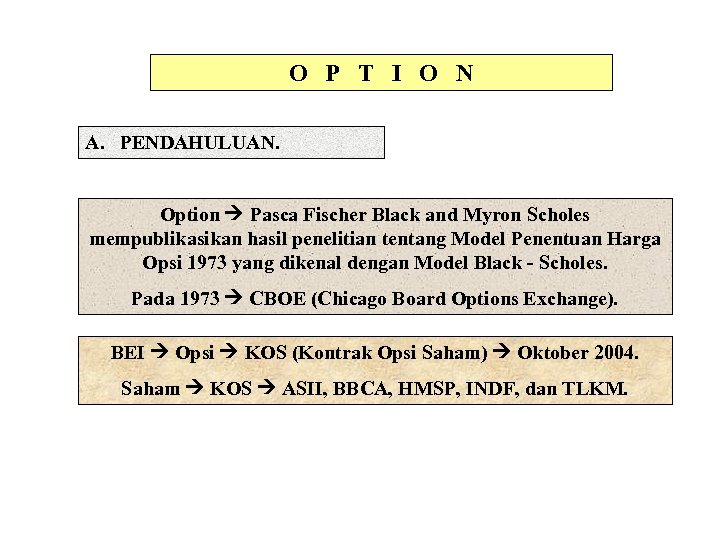O P T I O N A. PENDAHULUAN. Option Pasca Fischer Black and Myron Scholes mempublikasikan hasil penelitian tentang Model Penentuan Harga Opsi 1973 yang dikenal dengan Model Black - Scholes. Pada 1973 CBOE (Chicago Board Options Exchange). BEI Opsi KOS (Kontrak Opsi Saham) Oktober 2004. Saham KOS ASII, BBCA, HMSP, INDF, dan TLKM.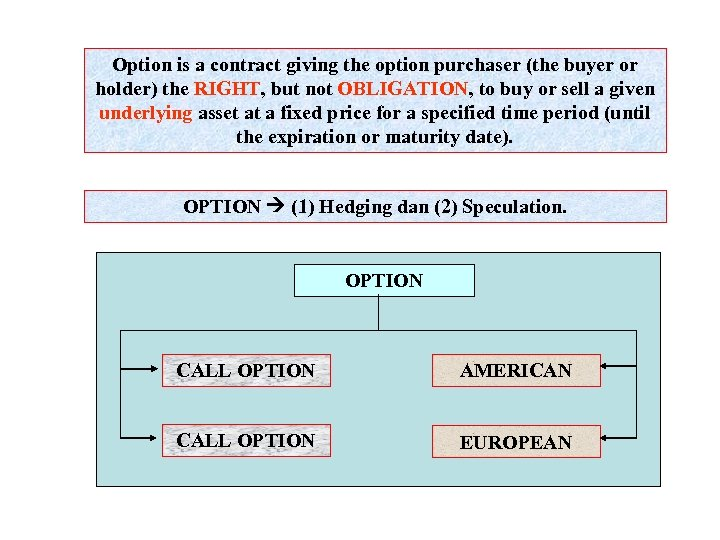Option is a contract giving the option purchaser (the buyer or holder) the RIGHT, but not OBLIGATION, to buy or sell a given underlying asset at a fixed price for a specified time period (until the expiration or maturity date). OPTION (1) Hedging dan (2) Speculation. OPTION CALL OPTION AMERICAN CALL OPTION EUROPEAN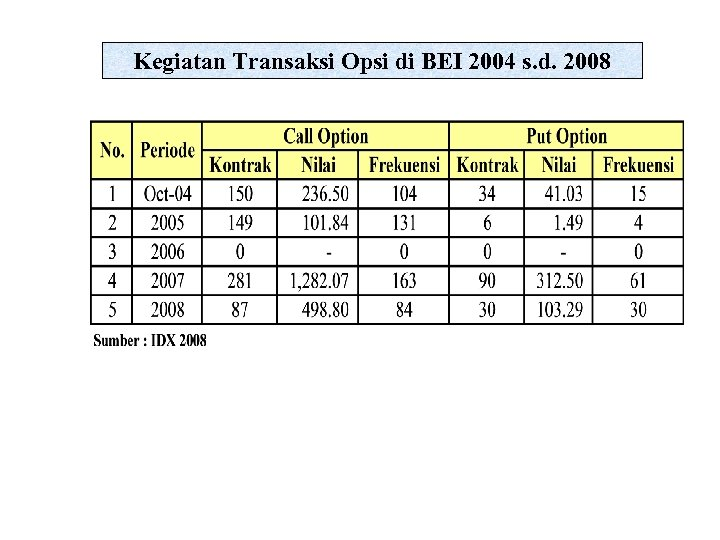Kegiatan Transaksi Opsi di BEI 2004 s. d. 2008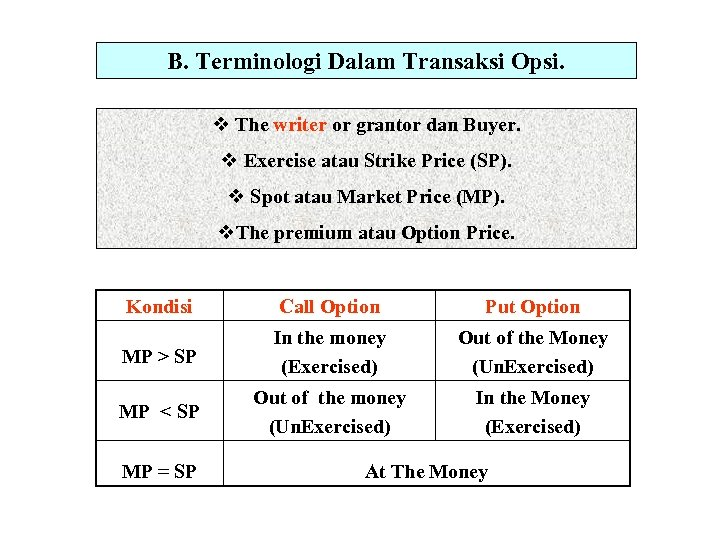B. Terminologi Dalam Transaksi Opsi. v The writer or grantor dan Buyer. v Exercise atau Strike Price (SP). v Spot atau Market Price (MP). v. The premium atau Option Price. Kondisi Call Option Put Option MP > SP In the money (Exercised) Out of the Money (Un. Exercised) MP < SP Out of the money (Un. Exercised) In the Money (Exercised) MP = SP At The Money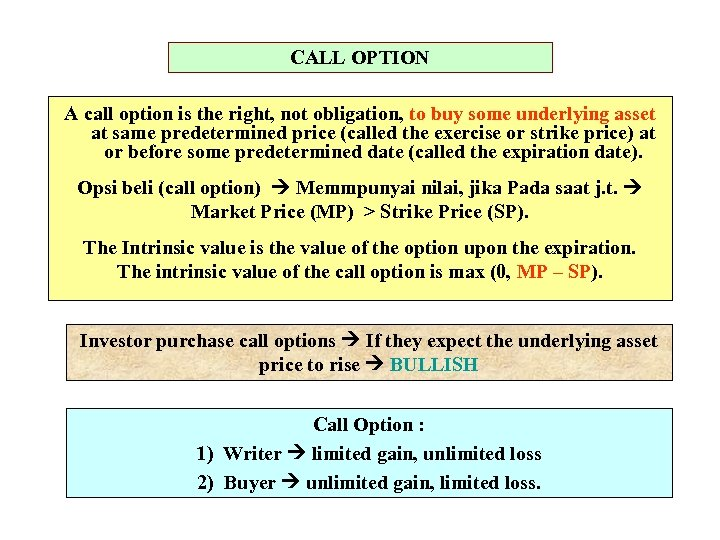CALL OPTION A call option is the right, not obligation, to buy some underlying asset at same predetermined price (called the exercise or strike price) at or before some predetermined date (called the expiration date). Opsi beli (call option) Memmpunyai nilai, jika Pada saat j. t. Market Price (MP) > Strike Price (SP). The Intrinsic value is the value of the option upon the expiration. The intrinsic value of the call option is max (0, MP – SP). Investor purchase call options If they expect the underlying asset price to rise BULLISH Call Option : 1) Writer limited gain, unlimited loss 2) Buyer unlimited gain, limited loss.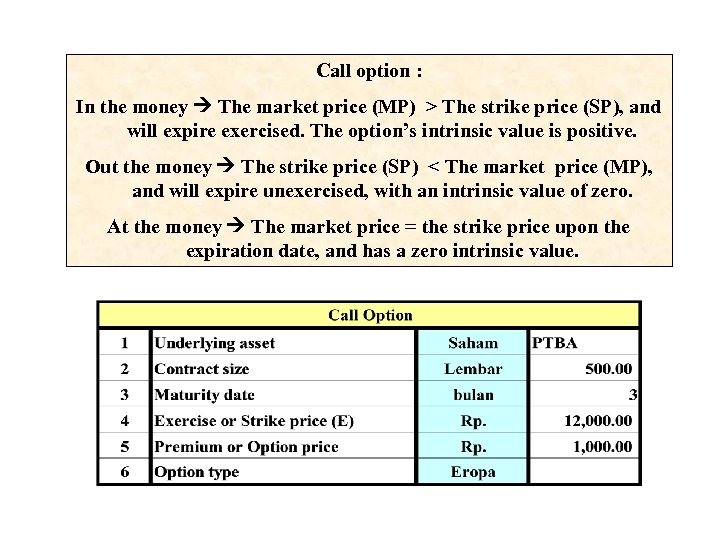Call option : In the money The market price (MP) > The strike price (SP), and will expire exercised. The option’s intrinsic value is positive. Out the money The strike price (SP) < The market price (MP), and will expire unexercised, with an intrinsic value of zero. At the money The market price = the strike price upon the expiration date, and has a zero intrinsic value.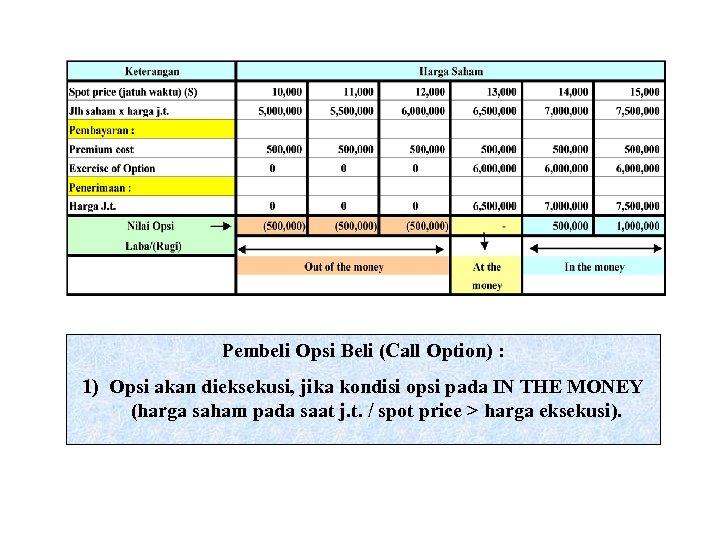Pembeli Opsi Beli (Call Option) : 1) Opsi akan dieksekusi, jika kondisi opsi pada IN THE MONEY (harga saham pada saat j. t. / spot price > harga eksekusi).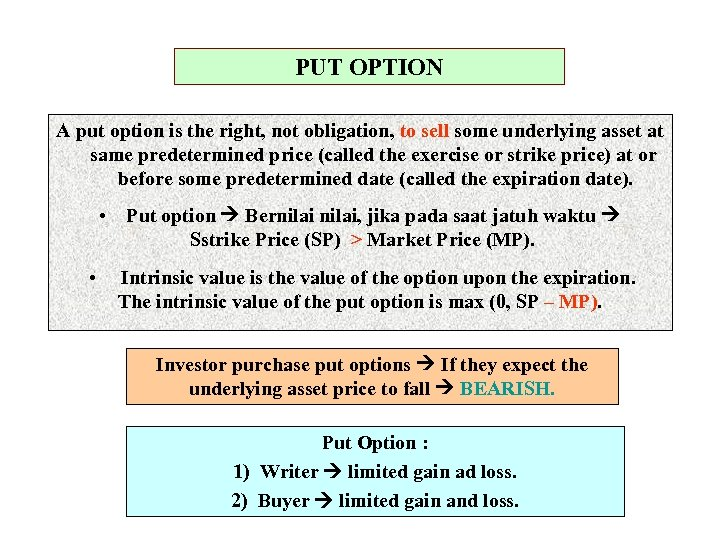PUT OPTION A put option is the right, not obligation, to sell some underlying asset at same predetermined price (called the exercise or strike price) at or before some predetermined date (called the expiration date). • Put option Bernilai, jika pada saat jatuh waktu Sstrike Price (SP) > Market Price (MP). • Intrinsic value is the value of the option upon the expiration. The intrinsic value of the put option is max (0, SP – MP). Investor purchase put options If they expect the underlying asset price to fall BEARISH. Put Option : 1) Writer limited gain ad loss. 2) Buyer limited gain and loss.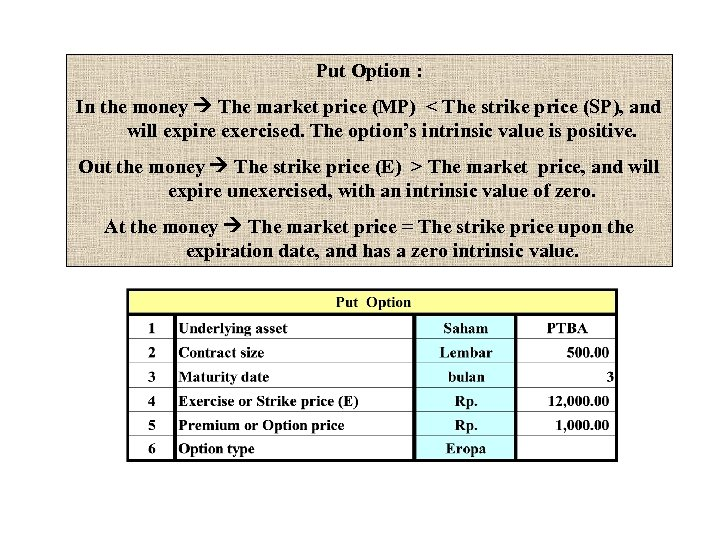Put Option : In the money The market price (MP) < The strike price (SP), and will expire exercised. The option’s intrinsic value is positive. Out the money The strike price (E) > The market price, and will expire unexercised, with an intrinsic value of zero. At the money The market price = The strike price upon the expiration date, and has a zero intrinsic value.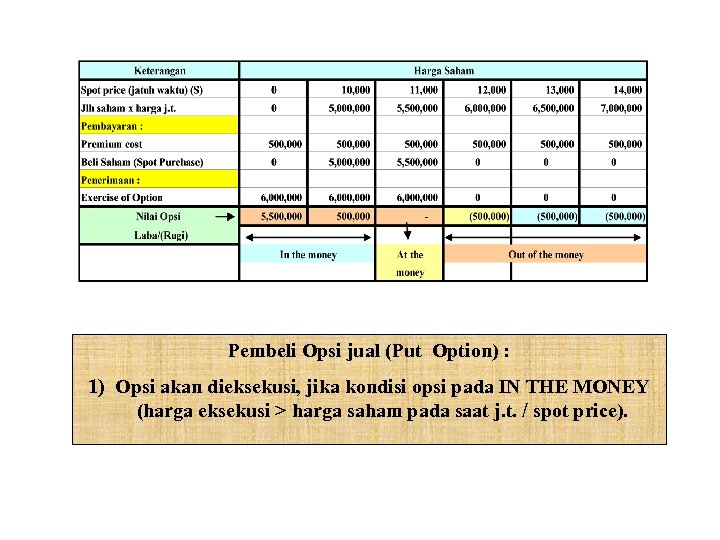Pembeli Opsi jual (Put Option) : 1) Opsi akan dieksekusi, jika kondisi opsi pada IN THE MONEY (harga eksekusi > harga saham pada saat j. t. / spot price).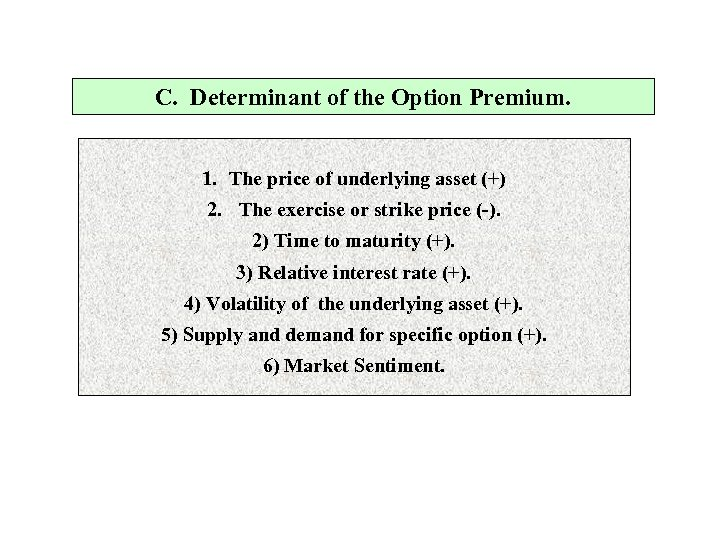C. Determinant of the Option Premium. 1. The price of underlying asset (+) 2. The exercise or strike price (-). 2) Time to maturity (+). 3) Relative interest rate (+). 4) Volatility of the underlying asset (+). 5) Supply and demand for specific option (+). 6) Market Sentiment.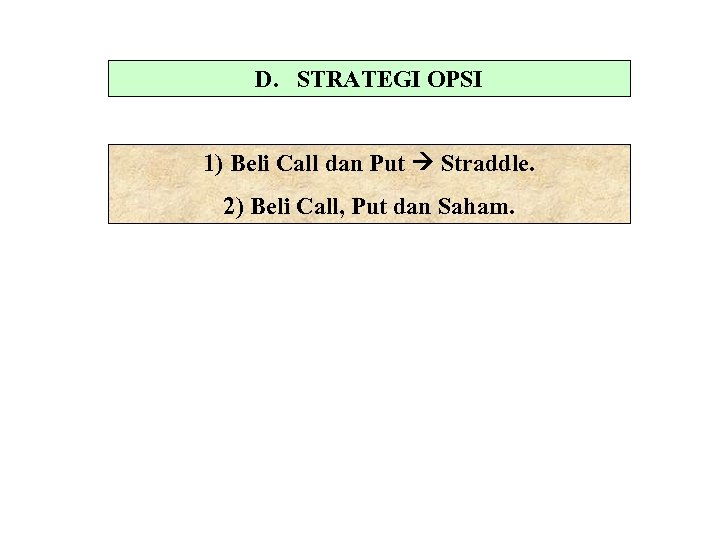D. STRATEGI OPSI 1) Beli Call dan Put Straddle. 2) Beli Call, Put dan Saham.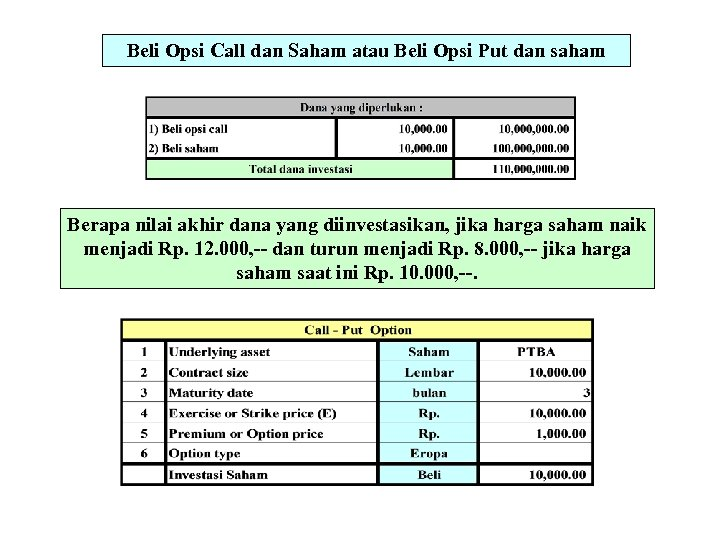Beli Opsi Call dan Saham atau Beli Opsi Put dan saham Berapa nilai akhir dana yang diinvestasikan, jika harga saham naik menjadi Rp. 12. 000, -- dan turun menjadi Rp. 8. 000, -- jika harga saham saat ini Rp. 10. 000, --.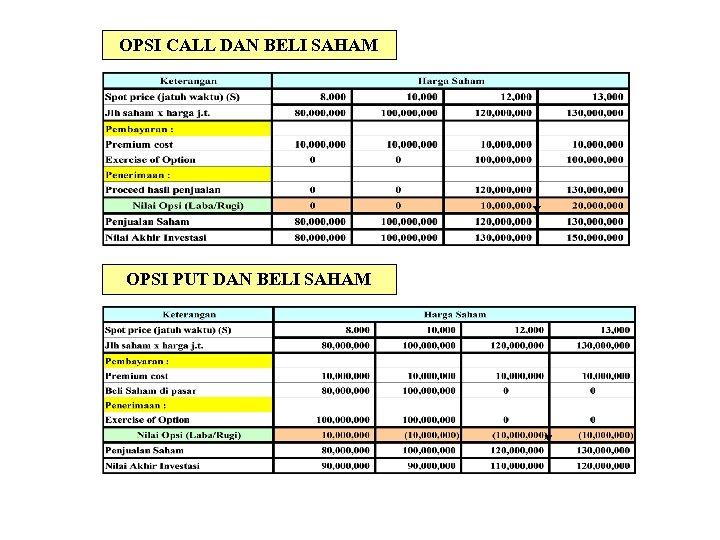OPSI CALL DAN BELI SAHAM OPSI PUT DAN BELI SAHAM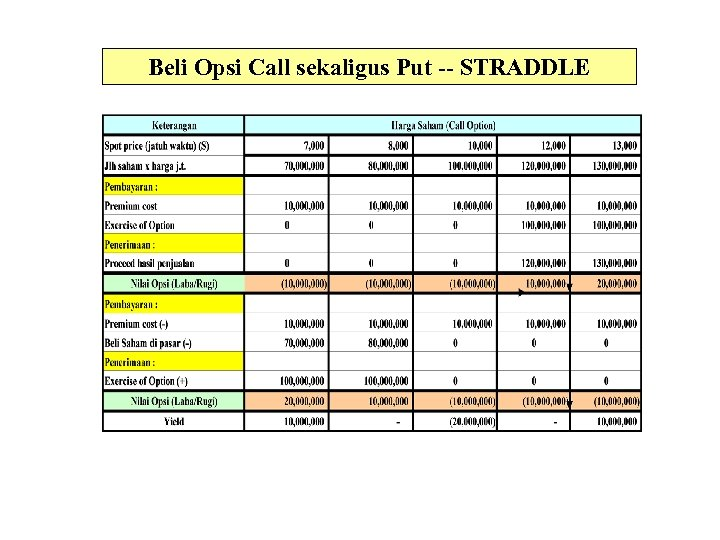Beli Opsi Call sekaligus Put -- STRADDLE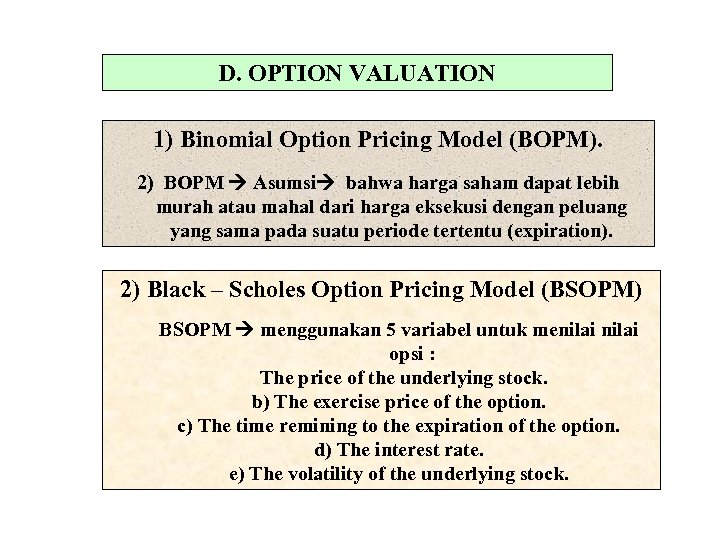D. OPTION VALUATION 1) Binomial Option Pricing Model (BOPM). 2) BOPM Asumsi bahwa harga saham dapat lebih murah atau mahal dari harga eksekusi dengan peluang yang sama pada suatu periode tertentu (expiration). 2) Black – Scholes Option Pricing Model (BSOPM) BSOPM menggunakan 5 variabel untuk menilai opsi : The price of the underlying stock. b) The exercise price of the option. c) The time remining to the expiration of the option. d) The interest rate. e) The volatility of the underlying stock.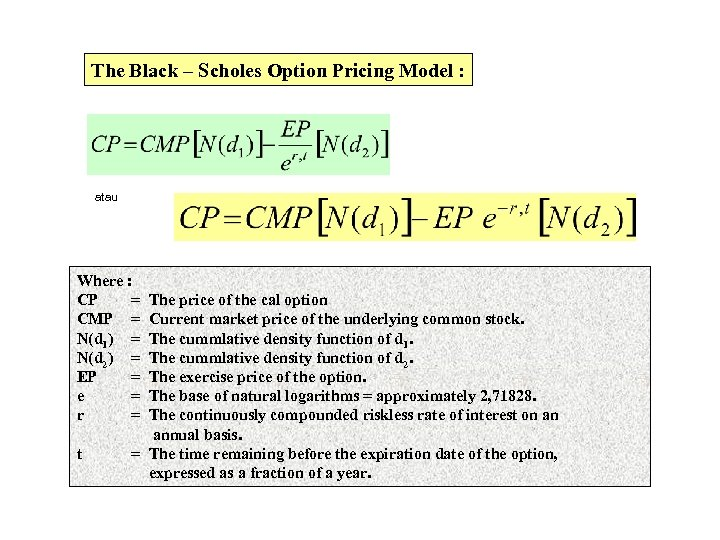The Black – Scholes Option Pricing Model : atau Where : CP = CMP = N(d 1) = N(d 2) = EP = e = r = t The price of the cal option Current market price of the underlying common stock. The cummlative density function of d 1. The cummlative density function of d 2. The exercise price of the option. The base of natural logarithms = approximately 2, 71828. The continuously compounded riskless rate of interest on an annual basis. = The time remaining before the expiration date of the option, expressed as a fraction of a year.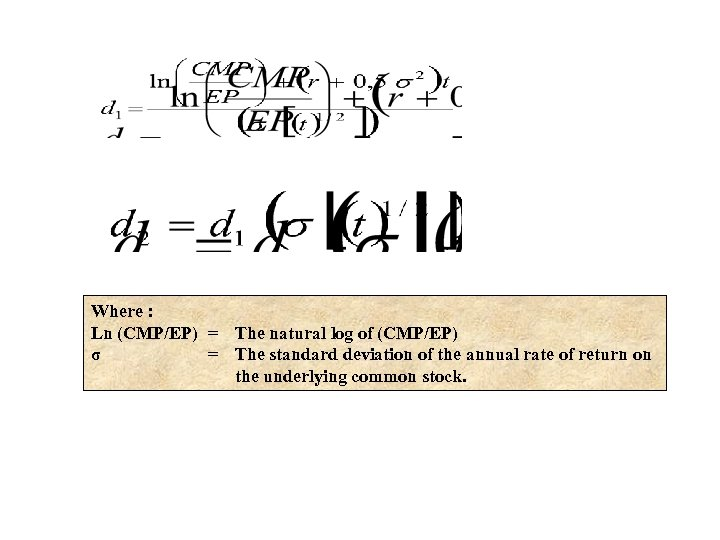Where : Ln (CMP/EP) = The natural log of (CMP/EP) σ = The standard deviation of the annual rate of return on the underlying common stock.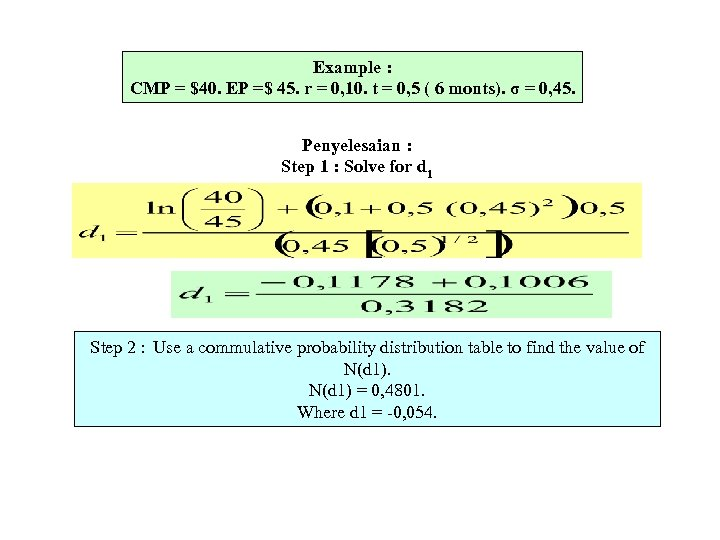Example : CMP = \$40. EP =\$ 45. r = 0, 10. t = 0, 5 ( 6 monts). σ = 0, 45. Penyelesaian : Step 1 : Solve for d 1 Step 2 : Use a commulative probability distribution table to find the value of N(d 1) = 0, 4801. Where d 1 = -0, 054.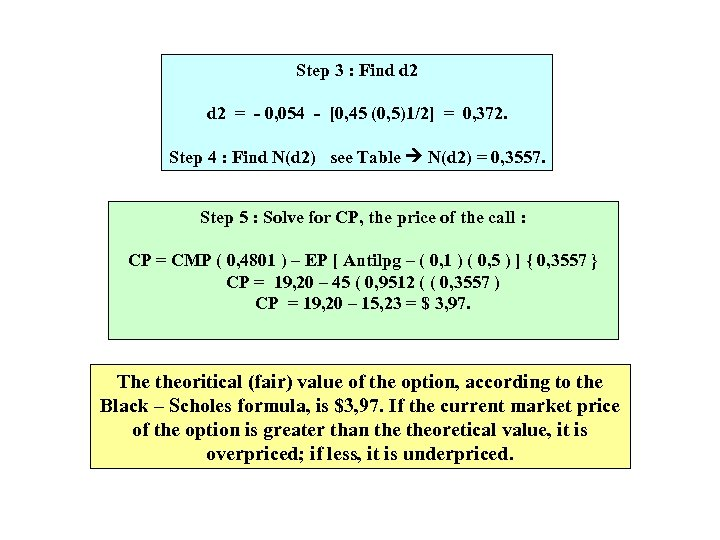Step 3 : Find d 2 = - 0, 054 - [0, 45 (0, 5)1/2] = 0, 372. Step 4 : Find N(d 2) see Table N(d 2) = 0, 3557. Step 5 : Solve for CP, the price of the call : CP = CMP ( 0, 4801 ) – EP [ Antilpg – ( 0, 1 ) ( 0, 5 ) ] { 0, 3557 } CP = 19, 20 – 45 ( 0, 9512 ( ( 0, 3557 ) CP = 19, 20 – 15, 23 = \$ 3, 97. The theoritical (fair) value of the option, according to the Black – Scholes formula, is \$3, 97. If the current market price of the option is greater than theoretical value, it is overpriced; if less, it is underpriced.